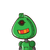# the three sides of a triangle are 150cm,120cm,200cm.find its area?also find the perpendicular on the largest side

the three sides of a triangle are 150cm,120cm,200cm.find its area?also find the perpendicular on the largest side

### 1 thought on “the three sides of a triangle are 150cm,120cm,200cm.find its area?also find the perpendicular on the largest side”

1.We can solve it by Heron’s formula.

So, semi perimeter will be,

s=

2

150+120+200

=235 cm

So,By herons’s formula:-

Area=

s(s−a)(s−b)(s−c)

,

where a=150 cm b=120 cm c=200 cm

By implying values:-

Area=

235(235−150)(235−120)(235−200)

Area=

235×85×115×35

Area=

80399375

Area=8966.57 cm

2